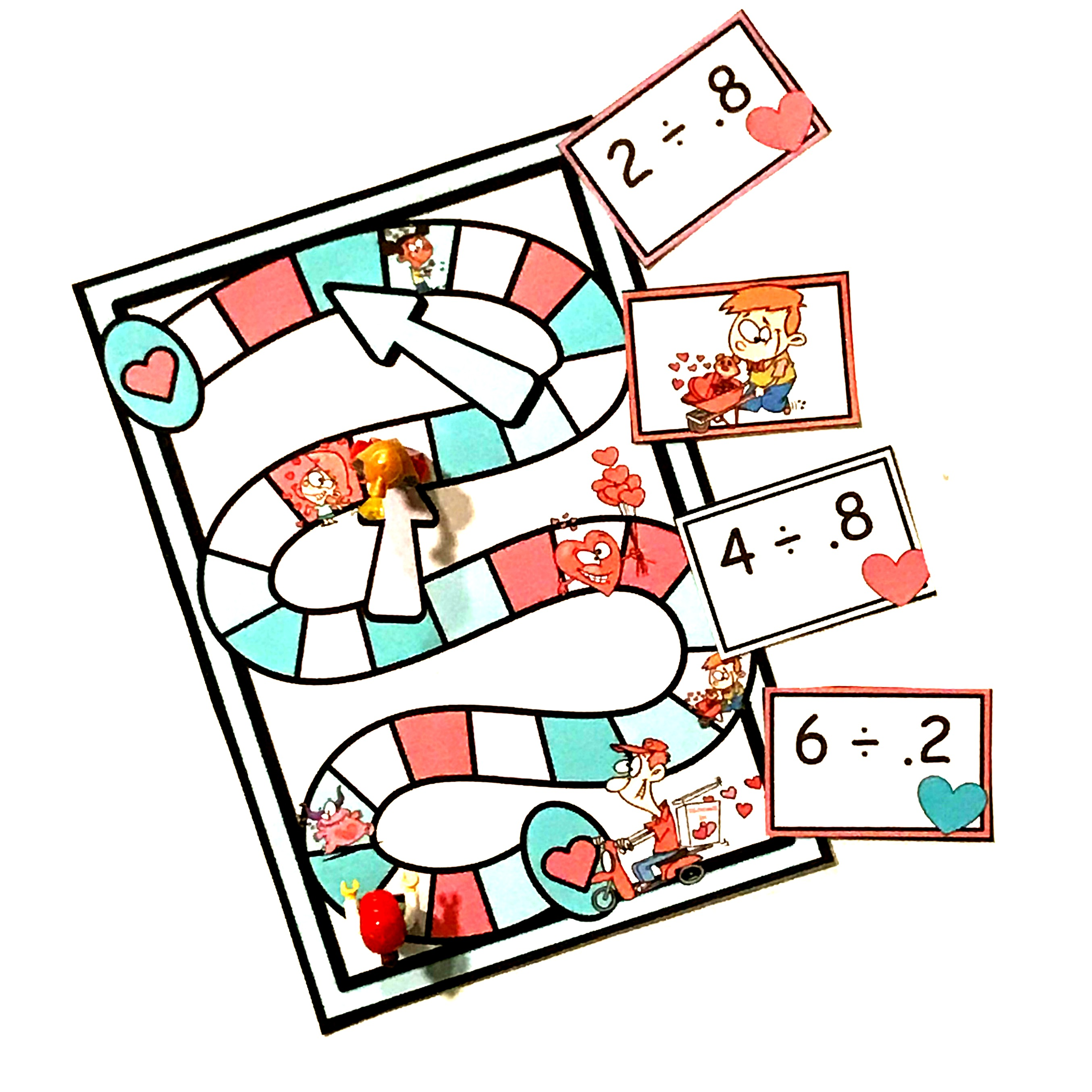# Free Game For Dividing Whole Numbers by Decimals

Ready to get to work on Dividing Whole Numbers by Decimals? Learn three different ways to teach this fun concept, and get a free game to practice it! Want more decimal activities? Then check out 40 Activities to Strengthen Decimal Sense.

If you read any of my work, you know that I’m a firm believer in hands-on lessons so that children understand the math behind an algorithm. Algorithms have their place. Seriously, I don’t want to be drawing 36 decimal grids to solve 36/.5……right?

But before we jump into teaching algorithms, I want to encourage you to teach dividing whole numbers by decimals with models.

These models will help our big kiddos to understand what is happening when we divide by a decimal. It will show them why we get a larger number when we divide with a decimal.

What? The answer is larger when we divide by a decimal?

Yes, it is….and our students need to see why this works.

## Using Decimal Grids

I love introducing decimals with decimal grids. It is such a simple way for children to be able to see that decimals are figured out based on tenths and hundredths. And it is also perfect for helping children see the relationship between fractions and decimals.

And once children understand decimal grids, we can add and subtract decimals or multiply and divide using this handy tool.

But for today, we are just going to look at dividing.

Let’s say I’m dividing two by five-tenths.

I will first color in two whole decimal grids.

Next, I divide those grids up so that there is five-tenths in each group.

How many groups do I have? Yep, four! Two divided by five-tenths is four.

And for some children seeing how you get an answer that is larger than the dividend is important. They will never understand why until they see it. And we always want our children to understand the why behind the algorithm.

These puzzles are a great way to get children analyzing decimal grids.

## Dividing Whole Numbers by Decimals Using Number Lines

Next, we jump to dividing on a number line.

I love number lines, but they should only be used with small dividends. (Otherwise, it will take up a lot of space and time!)

Let’s use the problem from above, and divide two by five-tenths again.

We begin by drawing a number line that has two wholes on it. In between the 0 and the 1, and the 1 and the 2, we will draw our tenths.

Now, once again we divide up the number line by five-tenths.

And once again we see that there are four equal parts.

This worksheet will give your kiddos a little number line practice.

## Dividing Whole Numbers by Decimals with Algorithms

Finally, we come to the algorithms. After having children play with number lines and decimal grids it is time to teach what we are most comfortable with.

Our children now have a good understanding of why we get a larger answer when we are dividing, so now we work in the algorithms.

Check out this video for step by step instructions.

And once they learn that algorithm. Here is a fun game for them to practice it. (Click on the large teal button at the end of the post to get it.)

With just a little cutting you have a game that adds a little fun to solving dividing whole numbers by decimals.

Dividing-By-Decimals-Game

This introduction to dividing decimals can be fun, and I hope the activities help you provide hands-on and fun instruction that builds decimal sense.

You’ve Got This,

Rachel